ď»ż
Reach Us+44-7482-875032
The Fast Assignment and Software Implementation of the Ultra-high Degree Earth’s Gravity Field Parameters

# Journal of Applied & Computational MathematicsOpen Access

All submissions of the EM system will be redirected to Online Manuscript Submission System. Authors are requested to submit articles directly to Online Manuscript Submission System of respective journal.

# The Fast Assignment and Software Implementation of the Ultra-high Degree Earth?s Gravity Field Parameters

Wei L1,2* and Zhang Z3
1Faculty of Geomatics and Geographic Information System, Lanzhou Jiaotong University, Lanzhou, P.R. China
2School of Geodesy and Geomatics, Wuhan University, Wuhan, P.R. China
3School of Mathematics and Physics, Lanzhou Jiaotong University, P.R. China
*Corresponding Author: Wei L, School of Mathematics and Physics, Lanzhou Jiaotong University, P.R. China, Tel: +86 2973619, Email: [email protected]

Received Date: Jul 17, 2017 / Accepted Date: Aug 16, 2018 / Published Date: Aug 21, 2018

Keywords: The gravity field; Ultra-high degree; Legendre function; Fast assignment

#### Introduction

The Earth gravitational potential is the basic function which characterizes the Earth’s gravity field, when we introduces the normal potential function properly, the disturbing potential function of the Earth’s gravitational field can be defined, the simple linear operator operation of the disturbing potential can export height anomalies, gravity anomalies, gravity disturbances, geoid undulations and deflections of the vertical, they all have important applications to the Earth's gravity field parameters . Modern Earth gravity field model gradually to the ultra-high degree development, for example, the EGM2008 model has been reached 2190 order, therefore it is of great significance to the application of the gravity field model to take advantage of ultra-high degree gravity field model to accurate gravity field parameters of any point on the earth outside space fast and stably [2-4].

The predecessors have done a lot of work on the Earth’s gravity field parameters from the theoretical to the practical calculation, they got a lot of models which is approximate to the calculation. From the international point of view, the parameter calculation model in the literature  can be up to 180 order, single point calculation time is about 0.48s, 0.46s. For higher degree parameter calculation, the computer will generate underflow messages. After recursive optimization, the Legendre function computing order can be up to 2700 order in Literature [6-8]. China mainly dedicated to the construction and application of the Earth’s gravity field model (also called the parameter calculation) as well as the fast solution which is related to the fully normalized of associating Legendre function and its derivatives [9-11].

This paper focuses on the construction of mathematical model, the implementation of the ultra-high degree and fast assignment (avoid some underflow messages), program design and software production as well as the numerical calculation and analysis of the results with measured data [12-15].

#### Mathematical Model

Because there are some certain analytic relationship between the Earth's geopotential, the gravitational potential and the centrifugal potential, and the Earth’s gravitational field is a harmonic field in the plastid outer earth . The gravitational potential V of Particle P(r,ϕ,λ) which satisfies the Laplace’s function, in the geocentric coordinate system of the Earth, the general solution is from the linear combination of the particular solution: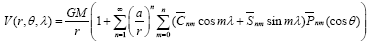(1)

In the above formula, γ is the geocentric radius , ϕ is the geocentric latitude, θ is the colatitude, and ϕ +θ = 90° , λ is the longitude, GM is the product of Newton’s gravitational constant G and the Earth’s total mass, a is the reference ellipsoid semi-major axis, n and m is the positive integer, and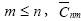and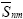is the fully normalized potential coefficients. In this formula, even degree zonal harmonic coefficient represents the actual gravitational potential and normal gravitational potential coefficient difference,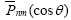is the fully normalized of associating Legendre function of n degree m order.

Because of the normal ellipsoid with respect to the symmetry axis, the gravitational potential solution has the following special form: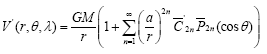(2)

Let W be the Earth's geopotential, U is the actual geopotential of normal ellipsoid, then the disturbance potential will be:

T =W −U = V −V ' (3)

Put in eqn. (1) and eqn. (2) respectively into expression in eqn. (3), when ΔT = 0 , we can get the general solution form of Laplace’s function of disturbance potential: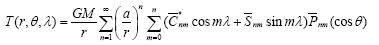(4)

In this equation,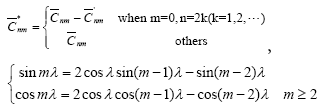(5)

The Spherical harmonic coefficients of expansion of the Earth’s geopotential (disturbance potential) model, referred to as the Earth's gravity field model, construction of this model is to determine a set of in eqn. (4) coefficients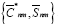.

According to Molodensky boundary conditions, for the gravity anomaly, we have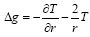, then: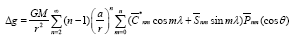(6)

Using the relationship between height anomalies ζ, gravity disturbances δg, deflections of the vertical (N-S component ξ and W-E component η ) and disturbance potential T, we can get the Spherical harmonic coefficients of expansion of height anomalies , gravity disturbances and deflections of the vertical, which is similar to formula in eqn. (6).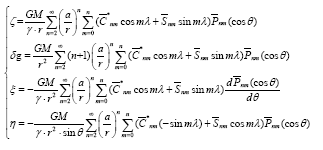(7)

In this equation, γ (r,ϕ ) is the normal gravity of Particle P(r,ϕ,λ ) [6,7].

#### The Stable Recursive Calculation of Ultra-High Degree Fully Normalized Of Associating Legendre Function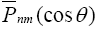As the Earth's gravity field model to the ultra-high degree direction, the implementation of gravity field parameter assignment which was based on the mathematical model cannot meet the requirement in precision and efficiency, namely the implementation of ultra-high degree and fast assignment. The calculation of associating Legendre function mainly uses the recursive algorithm [3,4,9-11], especially in the ultra-high degree disturbance potential functional and computational speed higher areas .

For the Spherical harmonic function sequence in the mathematical mode, this paper uses the following recursive formula: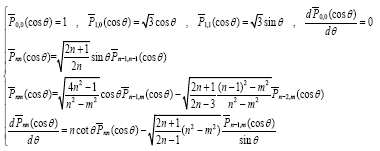(8)

And because of all kinds of recursive methods cannot avoid this situation, when near the poles [3,12],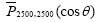approximate to 10-5000, and the general personal computer allows the numerical range of approximately between 10-310 and 10+310, then the recursive computation will produce underflow messages (The constraint of accuracy will make the calculated values are set to zero) . The program design of this paper, for an arbitrary calculation degree, the colatitude 0° ≤θ ≤ 180°, the introduction of the scaling factor Q(Q > 1, set Q = 1×10260). When we amplify Legendre function value, that is: Pnm (cosθ) ⋅Q , reduce the result of gravity field parameter, that is ζ ·Q-1. This ensures that the calculation of Legendre function value of arbitrary latitude to 2190 degree in the IEEE extended double precision floating-point data without data underflow phenomenon.

#### The Ultra-High Degree Gravity Field Parameter Assignment Software

In the software design, we use the class mechanism design method, make the parameter calculation, operation mode and graphic display design as an independent class , the parameters calculation is based on CComGra class among them, including the following functions: Read model geopotential coefficient(The software selected 2190 degree EGM2008 gravity field model coefficients ), ellipsoid parameter calculation, transformation of coordinate system, calculation of fully normalized of associating Legendre function, normal gravity calculation and calculation of gravity field parameters. The operation mode based on the MFC Dialog class, the input and output is in a window, operation information prompt and save the results. Graphical display is based on CSM Vector Show Ctrl class, support the twodimensional display output on the base map (The province boundary in China, the global boundary), including the following functions: the conversion of the logical coordinates and device coordinates, graphic plotting, point location information display, the map scaling and translation. In general, the software has the following characteristics:

a. Variable calculation type: The software uses the function parameter transfer function to design the multiple reference ellipsoidal type (WGS_84 or CGCS2000 or GRS80), model degree selection (This paper takes the 2190 degree calculation), point location input mode(Manual input or file read) in the same software, it reduces redundant processes and improve the efficiency of the software (Figure 1).

b. Less calculation: The key part adopted the fast algorithm, in the calculation of formula in eqns. (6) and (7), we all have the situation to solve the trigonometric sequence and spherical harmonic function. If we adopt the standard subroutine of internal machine, although the storage unit of intermediate results can be reduced, the calculation amount will increase. Especially in the model calculation of high degree case, if we repeatedly call the standard subroutine in the progress of n, m loop, the computing quantity will be too much. Therefore, in the calculation of trigonometric function sequence, adopting formula in eqn. (5) will save 10 times computation more than calling standard subroutine. For the calculation of spherical harmonic function sequence, we adopt the recursion formula in eqn. (8). If we store calculation results of trigonometric function and spherical harmonic function sequence for formula in eqns. (6) and (7) used in calculation, and a lot of computation will be saved (Figure 2).

c. High reliability: In precision, the data type of program adopt double precision type , its precision is 15~16 digit, values are in the range of 5.0 × 10324∼1.7 × 10328, after analysis and comparison, it fully meets the requirements of accuracy. In the software debugging, each computing function is strictly block tested to confirm the correctness of the calculation results. In addition, the gravity anomalies value from calculation and the related datum data will be compared to verify the calculation result of this software is correct (Figure 3).

d. Good portability: Computational software uses C++ language and is prepared based on the VS2008 development platform. As long as the machine internal and external memories meet the requirements of calculation software, calculation software can be easily ported to other machines.

#### Numerical Calculation and Analysis

The selected software of this paper using the coefficient of 2190 degree EGM2008 gravity field model potential coefficient , the test data comes from 492 GPS-leveling data of a region in China which were completed in 2004, there are height anomalies of high precision and high resolution in the test area, plane coordinate system belongs to the WGS_84 coordinate system, the elevation system belongs to National Elevation Datum in 1985.

Using the calculation software of ultra-high degree earth’s gravity field parameters, executing with step-by step:

a. Selecting the type of calculation parameters: height anomalies.

b. The type of the reference ellipsoid: WGS_84.

c. Calculated degree: 360, 720, 1080, 1440, 1800, 2160, 2190.

d. The input mode of point and location: the file is read into 492 points information (geodetic latitude, longitude, geodetic height).

e. Perform calculation.

To check the feasibility of mathematical model and the correctness of calculation results in this paper, compare the determined height anomalies from different degree calculation of model with the determined height anomalies of GPS-leveling data, the result is shown in Table 1.

 The truncation order time 360 720 1080 1440 1800 2160 2190 Max value 0.534 0.456 0.45 0.458 0.454 0.455 0.455 Min value -0.508 -0.415 -0.386 -0.387 -0.381 -0.376 -0.378 Average value 0.032 0.027 0.014 0.012 0.008 0.005 0.005 Standard deviation 0.172 0.15 0.143 0.143 0.142 0.142 0.142

Table 1: Statistical Information of height anomaly differences between different degree of Mathematical model of this paper and GPS-leveling (unit:m).

From the statistical results of Table 1, we can find the accuracy of height anomalies of mathematical model calculation in this paper, when the truncation order time increased from 360 degree to 1080 degree, the exaltation of accuracy is the most obvious, the extent is up to 17%. When the truncation degree is in the 1800 degree, 2160 degree and 2190 degree, the accuracy is 0.142 m.

The software is running in Windows environment, the computer configuration is Intel® CPU dual-core [email protected] GHz, the capacity of RAM is 1 GB. In computational efficiency, the computation time of 2190 degree single-point information gravity field parameters is in the range of 0.130s~0.185s.

#### Conclusion

This paper uses the existing model potential coefficients , combine the fast-recursive algorithm of high degree associating Legendre function, calculate the degree expansion of the earth’s disturbance potential Spherical harmonic series coefficients, and derive the related earth’s gravity field parameters through the mathematical model. We also use the C++ language, based on the VS2008 development platform, class mechanism, to conduct the compilation of algorithm, the debugging and packaging of function as well as software production. We obtain the following conclusions through numerical calculation and the analysis of results:

a. Introducing the method of scale factor in calculating the fully normalized of associating Legendre function, which can resolve the problems of digital underflow and stabilize recursive calculation up to 2190 degree, so as to implement the stable assignment of ultra-high degree gravity field parameters.

b. Optimizing calculation method to lower the complexity in the software implementation and reduce program-redundancy. It will make the computation time of the single-point information high degree gravity field parameters in the range of 0.130 s~0.185 s, and satisfy the correctness of the data.

c. To use the algorithm and software in this paper, obtain height anomalies through the calculation of EGM2008 model, compared with 492 GPS-leveling data and checked, when the model order is 2190 degree, the standard deviation is 0.142 m, it verifies the reliability of algorithm and software.

In summary, in determining the global gravity field parameters of high precision and high resolution, this paper presents a mathematical model and optimized program, appends ultra-high degree earth’s gravity field parameters calculation software with simplicity, efficiency, practicality and accuracy, which will do a great favor to further study the fine gravity field in the future.

#### References

Citation: Wei L, Zhang Z (2018) The Fast Assignment and Software Implementation of the Ultra-high Degree Earth’s Gravity Field Parameters. J Appl Computat Math 7: 419. DOI: 10.4172/2168-9679.1000419

Copyright: © 2018 Wei L, et al. This is an open-access article distributed under the terms of the Creative Commons Attribution License, which permits unrestricted use, distribution, and reproduction in any medium, provided the original author and source are credited.

Select your language of interest to view the total content in your interested language

##### Recommended Journals
Viewmore
###### Article Usage
• Total views: 897
• [From(publication date): 0-0 - Aug 24, 2019]
• Breakdown by view type
• HTML page views: 852
• PDF downloads: 45

## Post your commentCan't read the image? click here to refresh
###### Peer Reviewed Journals

Make the best use of Scientific Research and information from our 700 + peer reviewed, Open Access Journals

International Conferences 2019-20

Meet Inspiring Speakers and Experts at our 3000+ Global Annual Meetings

Top